Courses

# NCERT Solutions(Part- 3)- Understanding Quadrilaterals Class 8 Notes | EduRev

## Mathematics (Maths) Class 8

Created by: Full Circle

## Class 8 : NCERT Solutions(Part- 3)- Understanding Quadrilaterals Class 8 Notes | EduRev

The document NCERT Solutions(Part- 3)- Understanding Quadrilaterals Class 8 Notes | EduRev is a part of the Class 8 Course Mathematics (Maths) Class 8.
All you need of Class 8 at this link: Class 8

EXERCISE 3.3

Question 1. Given a parallelogram ABCD. Complete each statement along with the definition or property used.
(ii) ∠DCB = _____
(iii) OC = _____
(iv) m∠DAB + m∠CDA = _____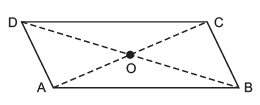Solution:
(i) AD = BC               [∵ Opposite sides are equal]
(ii) ∠DCB = ∠DAB   [∵ Opposite angles are equal]
(iii) OC = OA            [∵ Diagonals bisect each other]
(iv)m∠DAB + m∠CDA = 180°  [∵ Adjacent angles are supplementary]

Question 2. Consider the following parallelograms. Find the values of the unknowns x, y, z.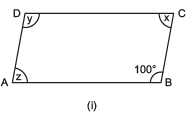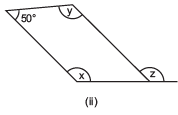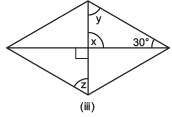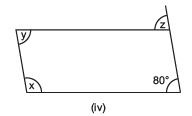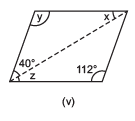Solution: (i) ∠y = 100°  [∵ Opposite angles of a parallelogram are equal.]
∵ Sum of interior angles of a parallelogram = 360°
∴  x + y + z + ∠B = 360°
or  x + 100° + z + 100° = 360°
or  x + z = 360° – 100° – 100° = 160°

But   x = z
∴ x = z = 160°/2 = 80°
Thus,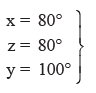(ii) ∵ Opposite angles are equal.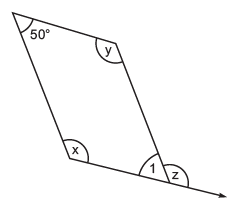∴ m∠1 = 50
Now, ∠1 + z = 180°  [Linear pair]
or  z = 180° – ∠1 = 180° – 50° = 130°
x + y + 50° + 50° = 360°

or  x + y = 360° – 50° – 50° = 260°
But  x = y
∴  x = y = 260°/2° = 130°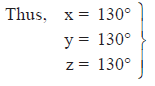(iii) ∵ Vertically opposite angles are equal,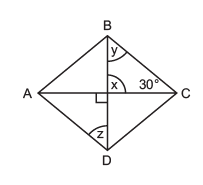∴ x = 90°

∵ Sum of the angles of a triangle = 180° ∴90° + 30° + y = 180°
or y = 180° – 30° – 90° = 60°

In the figure, ABCD is a parallelogram.
∴ AD || BC and BD is a transversal.
∴ y = z   [Alternate angles]
But y = 60°
∴ z = 60°
Thus, x = 90°, y = 60° and z = 60°.
(iv) ABCD is a parallelogram.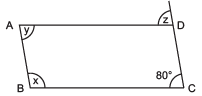∴ Opposite angles are equal.
∴ y = 80°

AB || CD and BC is a transversal.
∴ x + 80° = 180° [Interior opposite angles]
or   x = 180° – 80° = 100°

Again BC || AD and CD is a transversal,
∴ z = 80°   [Corresponding angles]

Thus, x = 100°, y = 80° and z = 80°
(v) ∵ In a parallelogram, opposite angles are equal.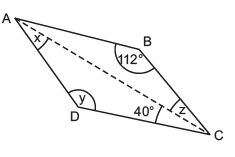∴  y = 112°
In Δ ACD,
x + y + 40° = 180°
x + 112° + 40° = 180°
∴  x = 180° – 112° – 40° = 28°
∵ AD || BC and AC is a transversal.
∴  x = z    [∵ Alternate angles are equal]

and z = 28°
Thus, x = 28°, y = 112° and z = 28°

Question 3. Can a quadrilateral ABCD be a parallelogram if
(i) ∠D + ∠B = 180°?
(ii) AB = DC = 8 cm, AD = 4 cm and BC = 4.4 cm?
(iii) ∠A = 70° and ∠C = 65°?
Solution: (i) In a quadrilateral ABCD,

∠A + ∠B = Sum of adjacent angles = 180°
∴  The quadrilateral may be a parallelogram but not always.

AB = DC = 8 cm
BC = 4.4 cm
∵ Opposite sides AB and BC are not equal.
It cannot be a parallelogram.

∠A = 70° and ∠C = 65°
∵ Opposite angles ∠A ≠ ∠C
∴ It cannot be a parallelogram.

Question 4. Draw a rough figure of a quadrilateral that is not a parallelogram but has exactly two opposite angles of equal measure.
Solution: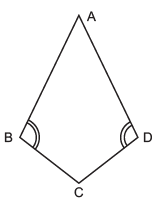In the adjoining figure, ABCD is not a parallelogram such that opposite angles –B and –D are equal. It is a kite.

Question 5. The measures of two adjacent angles of a parallelogram are in the ratio 3 : 2. Find the measure of each of the angles of the parallelogram.
Solution: Let ABCD be a parallelogram in which adjacent angles ∠A and ∠B are 3x and 2x respectively, since adjacent angles are supplementary.
∴  ∠A + ∠B = 180°
∴  3x + 2x = 180°

or  5x = 180°
or   x = 180°/5° = 36°
∴  ∠A = 3 x 36° = 108°
and B = 2 x 36° = 72°
∵ Opposite angles are equal.
∴   ∠D = ∠B = 72°
and ∠C= ∠A = 108°
∴   ∠A = 108°, ∠B = 72°, ∠C = 108° and ∠D = 72°

Question 6. Two adjacent angles of a parallelogram have equal measure. Find the measure of each of the angles of the parallelogram.

Solution: Let ABCD be a parallelogram such that adjacent angles ∠A = ∠B.
Since ∠A + ∠B = 180°
∴ A = ∠B = 180°/2 = 90°
Since, opposite angles of a parallelogram are equal.
∴  ∠A= ∠C = 90°
and ∠B= ∠D = 90°
Thus,  ∠A = 90°, ∠B = 90°,∠C = 90° and ∠D = 90°.

Question 7. The adjacent figure HOPE is a parallelogram. Find the angle measures x, y and z. State the properties you use to find them.
Solution:

y + z = 70° …(1)
In a triangle, exterior angles is equal to the sum of interior angles opposite.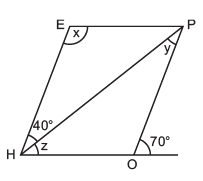∴ In Δ ∠HOP, ∠HOP = 180° -(y + z)
= 180° – 70°
= 110°
Now x = ∠HOP    [Opposite angles of a parallelogram are equal]
∴ x = 110°    EH || OP and PH is a transversal.
∴ y = 40°    [Alternate angles are equal]
From (1),   40° + z = 70°
∴   z = 70° – 40° = 30°

Thus, x = 110°, y = 40° and z = 30°

Question 8. The following figures GUNS and RUNS are parallelograms. Find x and y. (Lengths are in cm.)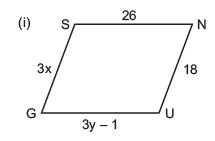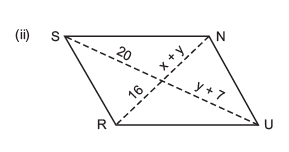Solution: (i) ∵ GUNS is a parallelogram.
∴ Its opposite sides are equal.
∴ GS = NU and SN = GU
or  3x = 18 and 26 = 3y – 1
Now 3x = 18
⇒   x = 18/3 = 6
3y – 1 = 26
⇒   y = 26 + 1/3 = 27/3 = 9

Thus, x = 6 cm and y = 9 cm
(ii) RUNS is a parallelogram and thus its diagonals bisect each other.
∴  x + y = 16 and 7 + y = 20

i.e. y = 20 – 7
or   y = 13
∴  From x + y = 16, we have
x + 13 = 16

or   x = 16 – 13 = 3

Thus, x = 3 cm and y = 13 cm.

Question 9. In the following figure, both RISK and CLUE are parallelogram. Find the value of x.
Solution: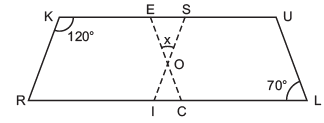RISK is a parallelogram.
∴  ∠R + ∠K = 180°     [∵ Adjacent angles of a parallelogram are supplementary]
or  ∠R + 120° = 180°
⇒  ∠R = 180° – 120° = 60°
But  ∠R and ∠S are opposite angles.
∴ ∠S = 60°
CLUE is also a parallelogram.
∴ Its opposite angles are equal.
∴ ∠E= ∠L = 70°

Now, in Δ ESO, we have
∠E + ∠S + x = 180°
∴  70° + 60° + x = 180°
or  x = 180° – 60° – 70°

⇒ x = 50

Question 10. Explain how this figure is a trapezium. Which of its two sides are parallel?°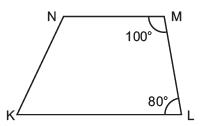Solution: Since, 100° + 80° = 180°
i.e. ∠M and ∠L are supplementary.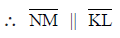[∵ If interior opposite angles along the transversal are supplementary]

Question 11. Find m∠C in the adjoining figure if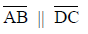.
Solution: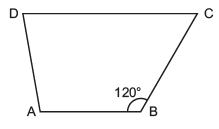∵ ABCD is a trapezium in whichand BC is a transversal.
∴   Interior opposite angles along BC are supplementary.
∴   m∠B + m∠C = 180°
or  m∠C = 180° – m∠B
∴   m∠C = 180° – 120°        [∵ ∠B = 120°]

or m∠C = 60°

Question 12. Find the measure of ∠P and ∠ S if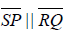in figure. (If you find m∠R, is there more than one method to find m∠P?)
Solution: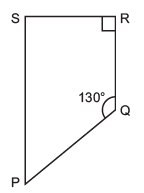PQRS is a trapezium such thatand PQ is a transversal.
∴  m∠P + m∠Q = 180°     [Interior opposite angles are supplementary]
or  m∠P + 130° = 180° or m∠P = 180° – 130° = 50°
Again SP || RQ and RS is a transversal.

∴ m∠S + m–R = 180°

or  m–S + 90° = 180°
∴ m∠S = 180° – 90° = 90°
Yes, using the angle sum property of a quadrilateral, we can find m∠P when m∠R is known.
∴ m∠P + m–Q + m∠R + m∠S = 360°
or  m∠P + 130° + 90° + 90° = 360°
or m∠P = 360° – 130° – 90° – 90° = 50°

## Mathematics (Maths) Class 8

212 videos|147 docs|48 tests

,

,

,

,

,

,

,

,

,

,

,

,

,

,

,

,

,

,

,

,

,

;